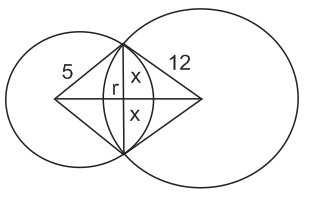# If the angle of intersection at a point where two circles with radii  and  intersects is , then the length (in cm) of their common chord is : Option 1)Option 2)Option 3)Option 4)Length of common chord =

Option 1)

Option 2)

Option 3)

Option 4)

Exams
Articles
Questions